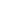Flat 50% off

Ends in

• JEE Main
•• Mathematics
• Back to Doubt Clearing

## Trigonometry doubtLet A and B denote the satements                            A : $\cos \alpha+\cos \beta+\cos \gamma=0$                            B : $\sin \alpha+\sin \beta+\sin \gamma=0$If $\cos (\beta-\gamma)+\cos (\gamma-\alpha)+\cos (\alpha-\beta)=-\frac{3}{2},$then (a) Both A and B are true(b)  Both A and B are false(c) A is true and B is false(d) A is false and B is true

### Asked By Vishal

Updated Fri, 31 May 2019 05:28 pm